Advertisement Remove all ads

# NCERT solutions for Class 11 Physics chapter 6 - Work, Energy and Power [Latest edition]

#### Chapters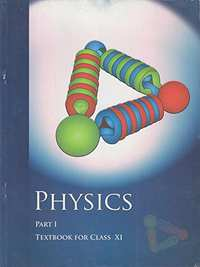Advertisement Remove all ads
Advertisement Remove all ads
Advertisement Remove all ads

## Chapter 6: Work, Energy and Power

Advertisement Remove all ads
Extra questions

### NCERT solutions for Class 11 Physics Chapter 6 Work, Energy and Power Extra questions

State if each of the following statements is true or false. Give reasons for your answer.

In an inelastic collision, the final kinetic energy is always less than the initial kinetic energy of the system.

[Pages 134 - 138]

### NCERT solutions for Class 11 Physics Chapter 6 Work, Energy and Power [Pages 134 - 138]

Q 2 | Page 134

A body of mass 2 kg initially at rest moves under the action of an applied horizontal force of 7 N on a table with coefficient of kinetic friction = 0.1. Compute the

(a) Work done by the applied force in 10 s

(b) Work done by friction in 10 s

(c) Work done by the net force on the body in 10 s

(d) Change in kinetic energy of the body in 10 s and interpret your results.

Q 3 | Page 134

Given in Figure  are examples of some potential energy functions in one dimension. The total energy of the particle is indicated by a cross on the ordinate axis. In each case, specify the regions, if any, in which the particle cannot be found for the given energy. Also, indicate the minimum total energy the particle must have in each case. Think of simple physical contexts for which these potential energy shapes are relevant.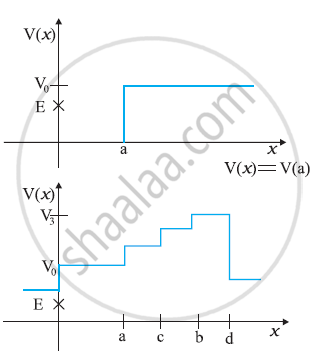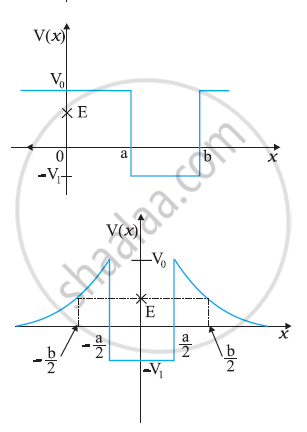Q 4 | Page 135

The potential energy function for a particle executing linear simple harmonic motion is given by V(x=kx2/2, where is the force constant of the oscillator. For k = 0.5 N m–1, the graph of V(x) versus is shown in Fig. 6.12. Show that a particle of total energy 1 J moving under this potential must ‘turn back’ when it reaches = ± 2 m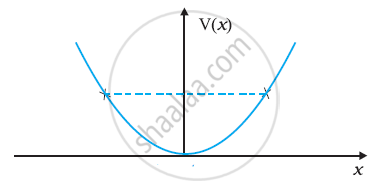Q 5.1 | Page 135

Answer the following:

The casing of a rocket in flight burns up due to friction. At whose expense is the heat energy required for burning obtained? The rocket or the atmosphere?

Q 5.2 | Page 135

Answer the following:

Comets move around the sun in highly elliptical orbits. The gravitational force on the comet due to the sun is not normal to the comet’s velocity in general. Yet the work done by the gravitational force over every complete orbit of the comet is zero. Why?

Q 5.3 | Page 135

Answer the following:

An artificial satellite orbiting the earth in very thin atmosphere loses its energy gradually due to dissipation against atmospheric resistance, however small. Why then does its speed increase progressively as it comes closer and closer to the earth?

Q 5.4 | Page 135

In Figure (i) the man walks 2 m carrying a mass of 15 kg on his hands. In Figure (ii), he walks the same distance pulling the rope behind him. The rope goes over a pulley, and a mass of 15 kg hangs at its other end. In which case is the work done greater ?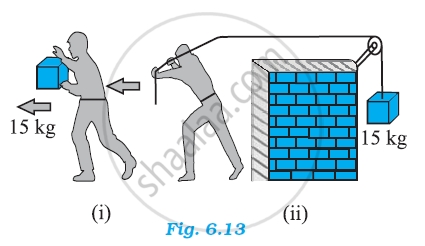Q 6.1 | Page 135

Underline the correct alternative:

When a conservative force does positive work on a body, the potential energy of the body increases/decreases/remains unaltered.

Q 6.2 | Page 135

Underline the correct alternative:

Work done by a body against friction always results in a loss of its kinetic/potential energy

Q 6.3 | Page 135

Underline the correct alternative:

The rate of change of total momentum of a many-particle system is proportional to the external force/sum of the internal forces on the system.

Q 6.4 | Page 135

Underline the correct alternative:

In an inelastic collision of two bodies, the quantities which do not change after the collision are the total kinetic energy/total linear momentum/total energy of the system of two bodies.

Q 7.1 | Page 135

State if each of the following statements is true or false. Give reasons for your answer.

In an elastic collision of two bodies, the momentum and energy of each body is conserved.

Q 7.2 | Page 135

State if each of the following statements is true or false. Give reasons for your answer.

Total energy of a system is always conserved, no matter what internal and external forces on the body are present.

Q 7.3 | Page 135

State if each of the following statements is true or false. Give reasons for your answer.

Work done in the motion of a body over a closed loop is zero for every force in nature.

Q 8.1 | Page 135

Answer carefully, with reasons:

In an elastic collision of two billiard balls, is the total kinetic energy conserved during the short time of collision of the balls (i.e. when they are in contact)?

Q 8.2 | Page 135

Answer carefully, with reasons:

Is the total linear momentum conserved during the short time of an elastic collision of two balls?

Q 8.3 | Page 136

Answer carefully, with reasons:

What are the answers to (a) and (b) for an inelastic collision?

Q 8.4 | Page 136

Answer carefully, with reasons:

If the potential energy of two billiard balls depends only on the separation distance between their centres, is the collision elastic or inelastic? (Note, we are talking here of potential energy corresponding to the force during collision, not gravitational potential energy).

Q 9 | Page 136

A body is initially at rest. It undergoes one-dimensional motion with constant acceleration. The power delivered to it at time is proportional to

1) t^(1/2)

2) t

3)t^(3/2)

4)t^2

Q 10 | Page 136

A body is moving unidirectionally under the influence of a source of constant power. Its displacement in time t is proportional to

1)t^(1/2)

3) t

3) c^2

Q 11 | Page 136

A body constrained to move along the z-axis of a coordinate system is subject to a constant force F given by F = -hati+2hatj+3hatkN Where hati,hatj,hatj are unit vectors along the x-, y- and z-axis of the system respectively. What is the work done by this force in moving the body a distance of 4 m along the z-axis ?

Q 12 | Page 136

An electron and a proton are detected in a cosmic ray experiment, the first with kinetic energy 10 keV, and the second with 100 keV. Which is faster, the electron or the proton? Obtain the ratio of their speeds. (electron mass = 9.11 × 10–31 kg, proton mass = 1.67 ×10–27 kg, 1 eV = 1.60 × 10–19 J).

Q 13 | Page 136

A rain drop of radius 2 mm falls from a height of 500 m above the ground. It falls with decreasing acceleration (due to viscous resistance of the air) until at half its original height, it attains its maximum (terminal) speed, and moves with uniform speed thereafter. What is the work done by the gravitational force on the drop in the first and second half of its journey? What is the work done by the resistive force in the entire journey if its speed on reaching the ground is 10 m s–1?

Q 14 | Page 136

A molecule in a gas container hits a horizontal wall with speed 200 m s–1 and angle 30° with the normal, and rebounds with the same speed. Is momentum conserved in the collision? Is the collision elastic or inelastic?

Q 15 | Page 136

A pump on the ground floor of a building can pump up water to fill a tank of volume 30 m3 in 15 min. If the tank is 40 m above the ground, and the efficiency of the pump is 30%, how much electric power is consumed by the pump?

Q 16 | Page 136

Two identical ball bearings in contact with each other and resting on a frictionless table are hit head-on by another ball bearing of the same mass moving initially with a speed V. If the collision is elastic, which of the following figure is a possible result after collision?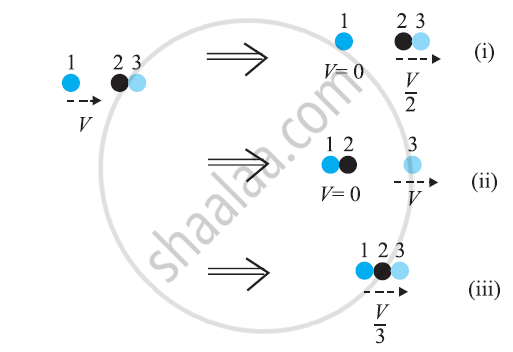Q 17 | Page 137

The bob A of a pendulum released from 30° to the vertical hits another bob B of the same mass at rest on a table as shown in Fig. How high does the bob A rise after the collision? Neglect the size of the bobs and assume the collision to be elastic.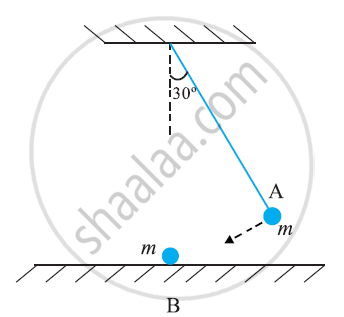Q 18 | Page 137

The bob of a pendulum is released from a horizontal position. If the length of the pendulum is 1.5 m, what is the speed with which the bob arrives at the lowermost point, given that it dissipated 5% of its initial energy against air resistance?

Q 19 | Page 137

A trolley of mass 300 kg carrying a sandbag of 25 kg is moving uniformly with a speed of 27 km/h on a frictionless track. After a while, sand starts leaking out of a hole on the floor of the trolley at the rate of 0.05 kg s–1. What is the speed of the trolley after the entire sand bag is empty?

Q 20 | Page 137

A body of mass 0.5 kg travels in a straight line with velocity v = ax3/2where a = 5 m1/2 s-1. What is the work done by the net force during its displacement from = 0 to = 2 m?

Q 21 | Page 137

The blades of a windmill sweep out a circle of area A. (a) If the wind flows at a velocity perpendicular to the circle, what is the mass of the air passing through it in time t?(b) What is the kinetic energy of the air? (c) Assume that the windmill converts 25% of the wind’s energy into electrical energy, and that = 30 m2= 36 km/h and the density of air is 1.2 kg m–3. What is the electrical power produced?

Q 22 | Page 137

A person trying to lose weight (dieter) lifts a 10 kg mass, one thousand times, to a height of 0.5 m each time. Assume that the potential energy lost each time she lowers the mass is dissipated, (a) How much work does she do against the gravitational force? (b) Fat supplies 3.8 x 107J of energy per kilogram which is converted to mechanical energy with a 20% efficiency rate. How much fat will the dieter use up?

Q 23 | Page 137

A family uses 8 kW of power. (a) Direct solar energy is incident on the horizontal surface at an average rate of 200 W per square meter. If 20% of this energy can be converted to useful electrical energy, how large an area is needed to supply 8 kW? (b) Compare this area to that of the roof of a typical house.

Q 24 | Page 137

A bullet of mass 0.012 kg and horizontal speed 70 m s–1 strikes a block of wood of mass 0.4 kg and instantly comes to rest with respect to the block. The block is suspended from the ceiling by means of thin wires. Calculate the height to which the block rises. Also, estimate the amount of heat produced in the block.

Q 25 | Page 137

Two inclined frictionless tracks, one gradual and the other steep meet at A from where two stones are allowed to slide down from rest, one on each track . Will the stones reach the bottom at the same time? Will they reach there with the same speed? Explain. Given θ1 = 30°, θ2 = 60°, and = 10 m, what are the speeds and times taken by the two stones?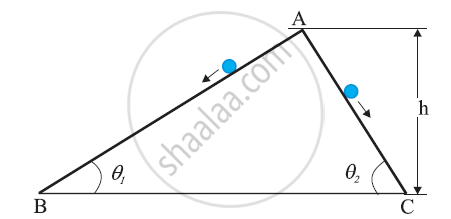Q 26 | Page 138

A 1 kg block situated on a rough incline is connected to a spring of spring constant 100 N m–1as shown in Fig. The block is released from rest with the spring in the unstretched position. The block moves 10 cm down the incline before coming to rest. Find the coefficient of friction between the block and the incline. Assume that the spring has a negligible mass and the pulley is frictionless.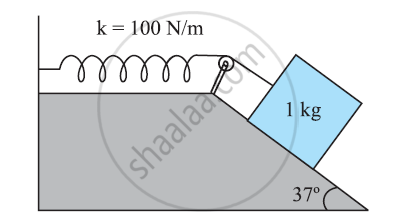Q 27 | Page 138

A bolt of mass 0.3 kg falls from the ceiling of an elevator moving down with an uniform speed of 7 m s–1. It hits the floor of the elevator (length of the elevator = 3 m) and does not rebound. What is the heat produced by the impact? Would your answer be different if the elevator were stationary?

Q 28 | Page 138

A trolley of mass 200 kg moves with a uniform speed of 36 km/h on a frictionless track. A child of mass 20 kg runs on the trolley from one end to the other (10 m away) with a speed of 4 m s–1 relative to the trolley in a direction opposite to the its motion, and jumps out of the trolley. What is the final speed of the trolley? How much has the trolley moved from the time the child begins to run?

Q 29 | Page 138

Which of the following potential energy curves in Fig. cannot possibly describe the elastic collision of two billiard balls? Here r is distance between centres of the balls.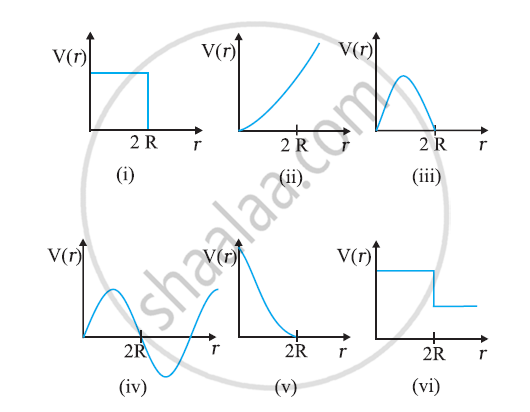Q 30 | Page 138

Consider the decay of a free neutron at rest : n → p + e

Show that the two-body decay of this type must necessarily give an electron of fixed energy and, therefore, cannot account for the observed continuous energy distribution in the β-decay of a neutron or a nucleus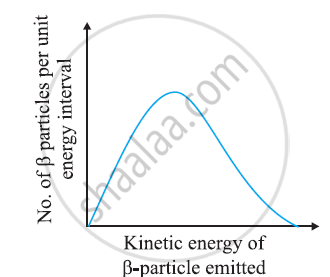## Chapter 6: Work, Energy and Power## NCERT solutions for Class 11 Physics chapter 6 - Work, Energy and Power

NCERT solutions for Class 11 Physics chapter 6 (Work, Energy and Power) include all questions with solution and detail explanation. This will clear students doubts about any question and improve application skills while preparing for board exams. The detailed, step-by-step solutions will help you understand the concepts better and clear your confusions, if any. Shaalaa.com has the CBSE Class 11 Physics solutions in a manner that help students grasp basic concepts better and faster.

Further, we at Shaalaa.com provide such solutions so that students can prepare for written exams. NCERT textbook solutions can be a core help for self-study and acts as a perfect self-help guidance for students.

Concepts covered in Class 11 Physics chapter 6 Work, Energy and Power are Introduction of Work, Energy and Power, Notions of Work and Kinetic Energy: the Work-Energy Theorem, Kinetic Energy, Work Done by a Constant Force and a Variable Force, The Concept of Potential Energy, The Conservation of Mechanical Energy, Potential Energy of a Spring, Various Forms of Energy : the Law of Conservation of Energy, Power, Concept of Collisions, Non - Conservative Forces - Motion in a Vertical Circle, Concept of Work.

Using NCERT Class 11 solutions Work, Energy and Power exercise by students are an easy way to prepare for the exams, as they involve solutions arranged chapter-wise also page wise. The questions involved in NCERT Solutions are important questions that can be asked in the final exam. Maximum students of CBSE Class 11 prefer NCERT Textbook Solutions to score more in exam.

Get the free view of chapter 6 Work, Energy and Power Class 11 extra questions for Class 11 Physics and can use Shaalaa.com to keep it handy for your exam preparation

Advertisement Remove all ads
Share
Notifications

View all notifications

Forgot password?